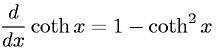Equations > Calculus > Differentiation/Differentals > Derivative of Hyperbolic Cotangent

### Derivative of Hyperbolic CotangentLatex Code:

MathML Code:

 $\fracd\mathrm{dx}\mathrm{coth}x=1-{\mathrm{coth}}^{2}x$

MathType 5.0: